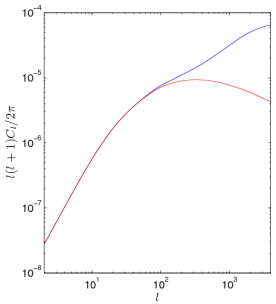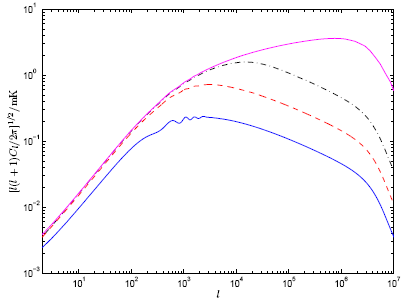CAMB sources: for number counts, lensing and dark-age 21cm power spectra
plus thermal history, perturbed recombination, CMB cross-correlations

##New: CAMB sources is now integrated with CAMB and functions available from python. See the main and python examples from Box 36 in the sample notebook.

Features:
• Dark age 21cm angular power spectra and transfer functions
• Angular power spectra for low-redshift source number counts (including redshift distortions and lensing)
• Lensing convergence power spectra from given window function (default: Gaussian)
• Use HALOFIT for non-linear corrections
• Various enhancements from CAMB for calculating high-l lensing potential and power spectra
• All cross-correlation power spectra (sources with themselves and with CMB)
• Full calculation and Limber approximation
• Baryon sound speed computed using evolved matter temperature perturbation
(hence transfer function more accurate than CAMB or CMBFAST on very small scales)
• Use third-order perturbation theory to calculate non-linear CDM power (and estimate 21cm) at high redshift (use second_PT instead of Halofit)
• Sharp frequency windows for 21cm (via get_transfer=T)
• Calculation of perturbed recombination for accurate gas temperature perturbations and small scale baryons
• Inclusion of self-absorption terms, baryon velocity divergence during perturbed recombination
• Improved treatment of count power spectra, including potential terms; .ini options to calculate various parts separately; separate function for physical background source distribution
• (Apr 11) efficient lensing convergence power spectra to small scales. Output of full cross-covariance matrix, including polarization, CMB lensing and any sources. Full openmp parallelization. Added Matlab plotting/reading scripts.
• NEW: (Nov 13) Improved handling of Limber approximation, including for full cross-correlations and magnification bias. Updates to match latest CAMB version as closely as possible, including updated Halofit from (significant increase in small-scale non-linear power) and CMB accuracy changes.
The paper for the counts calculation is , and the main reference for the 21cm results is ().

Download the . This version November 2013. The code is also available via CAMB_sources branch of CAMB's git repository.

See CosmoCoffee for support.

This code is based on CAMB which is based on CMBFAST which is partly based on COSMICS. It uses RECFAST for the ionization history.

Limitations:
• Not nearly as well tested and robust as main CAMB code
• Does not work for non-flat models
• Many non-standard parameter variations untested
• Accuracy not well quantified; also limited by ionization history and non-linear correction accuracy
• Not tested for compatibility with CosmoMC.
• 2nd order lensing effect of 21cm not included (though easy modification)
• Does not work with very narrow redshift window functions (use transfer_21cm_cl instead)
• Generating 21cm with narrow but not-sharp redshift window (0.01Mhz) can use > 1GB memory.

Usage

If you are familiar with CAMB this should be quite familiar. The main parameters are in the params.ini file - see the comments in this file for description. Sample files params_lensing.ini and params_counts.ini are also provided to give typical settings for lensing and number count results. Install and run e.g. like this:
• Edit the Makefile for your f90 compiler's options
• Run make
• Run ./camb params_lensing.ini (or ./camb params_counts.ini, ./camb params_21cm.ini)
• Make sure your result is stable to changes in the accuracy_boost, l_max_scalar and Kmax_Boost parameters, and variations in the Limber approximation options (e.g. on/off, changing limber_phiphi). Numerical accuracy for all possible combinations of cross-correlation spectra is not guaranteed with default settings.

Method

At low L the results are fully spherical and calculated by direct integration of source functions against spherical Bessel functions. The code assumes you have a finite-width window function (specified in modules.f90, implemented as a Gaussian in redshift by default), and integrals are integrated by parts (as in CAMB) so the window functions should be smooth. On small scales results can be calculated accurately much faster using the Limber approximation if you have set limber_windows = T, with the full spherical result being used at lower L. Limber can also be used for counts window functions on scales much narrower than the window width; the code tries to guess the appropriate scale at which to switch. Cross-correlation spectra should work as longer the domains of validity of the Limber approximation overlap. The limber_phiphi input parameter specifies the Limber L to use for the CMB lensing potential, and Limber is always switched for other window functions at higher L (so decrease limber_phiphi to use Limber to lower L, which is often accurate except for e.g. cross-correlation with the CMB temperature).

Output

Set the scalar_covariance_output_file to output a file with all of the auto and cross power spectra (by default _scalCovCls.dat). The (partially redundant) format of this file is columns of the form
`L C_11 C_12... C_1n C_21 C_22 .... C_nn`
where C_{ij} is L(L+1)CijL/2pi and the fields are ordered T, E, Phi, win_1, win_2..., where Phi is the CMB lensing potential. The power spectra are unlensed and dimensionless.

There are some supplied plotting scripts in ./python folder, e.g. see covCompare.py for a sample script to plot a matrix results in the _scalCovCls.dat file. You can also use the supplied plotWindowCl MatLab script e.g.

```plotWindowCl('test_scalCovCls.dat',1,2)
```
plots the angular cross-spectrum of the first and second window functions (CMB lensing potential is index 0,E index -1, and T index -2). You can also plot comparisons of multiple files with same L ranges (useful for testing numerical stability with different accuracy settings), e.g.
```plotWindowCl({'test_scalCovCls.dat','test2_scalCovCls.dat'},1,2)
```
Transfer functions are the same as in Buying metacam online canada, except when you are generating 21cm power spectrum in which case the matter power spectrum file becomes that for the monopole source, and the columns in the transfer function output file are
`k/h Delta_CDM/k^2 Delta_b/k^2 Monopole_source/k^2 v_newtonian/k^2 Delta_Tg/k^2 Delta_tot/k^2`
where all quantities are in the synchronous gauge.
scalCls file
Angular power spectra are also output to output_root_scalCls.dat. The columns are in the more confusing but less redundant form as used by earlier versions of CAMB sources:
`l C_TT C_EE C_TE C_Phi C_PhiT C_win_1 C_win_2 ... C_Win_T1 C_Win_T2 .... C_win1_win2 ...`
Here all C_l are l(l+1)C_l/2pi except for C_Phi and C_PhiT which are C_Phi= l^4 C_l_phi where C_l_phi is the (CMB) lensing potential power spectrum and C_PhiT = l^3 C_l_phiT. So for two window functions, columns 7 and 8 are their auto-spectra, and 11 is their cross-spectrum.

Non-linear evolution

By default compiles with Halofit for non-linear corrections at low redshift. Supplied code second_PT.f90 (not tested recently) instead uses third-order Newtonian perturbation theory. Change NONLINEAR in the Makefile to use this instead. Note that Halofit gives non-linear power spectra. second_PT instead gives just the non-linear correction (which is much smaller than the linear term at high redshift).